# Congruent Figures

Congruent figures are geometric figures that have the same shape and size. That is, if you can transform one figure into another figure by a sequence of translations , rotations , and/or reflections , then the two figures are congruent.

If the figures are polygons, then they are congruent if all the corresponding sides and corresponding angles are congruent.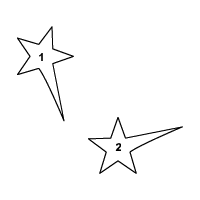Consider the polygon shown.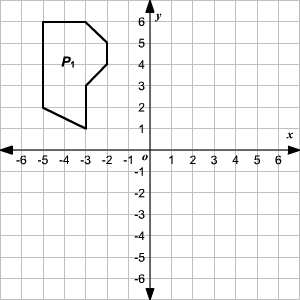Reflect the polygonabout the-axis.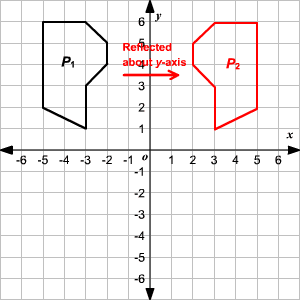The polygonsandare congruent figures. That is,.

Now, rotate the polygonbyabout the origin.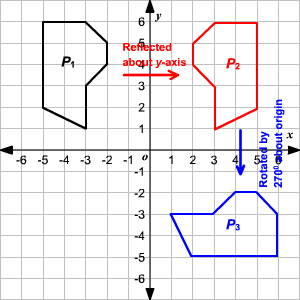Again,.

By the transitive property of congruence,.

Therefore, the polygon obtained by the transformations is congruent to the initial polygon.

Here, the hexagonis congruent to hexagon.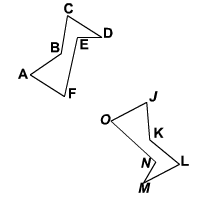Note that the order in which you write the vertices matters; for instance, hexagonis not congruent to hexagon.

Also see congruent triangles .